# Get First Word in Excel & Google Sheets

This tutorial will demonstrate how to extract the first word from a cell in Excel & Google Sheets.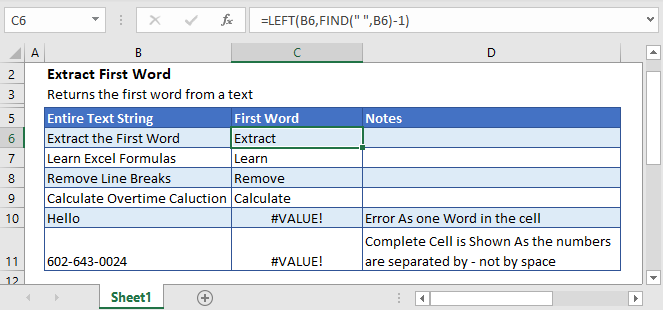## Extract First Word From a Text

To extract the first word from a cell, we can use a formula containing the LEFT and FIND functions.

``=LEFT(B3,FIND(" ", B3)-1)``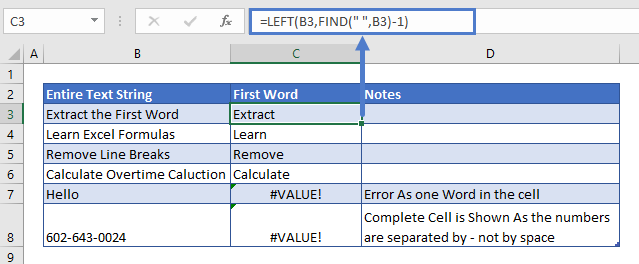Let’s delve into the more details of the functions used in the above formula.

### The FIND Function

We used the FIND function to get the position of the first occurrence of space in the text. By subtracting 1 from this, we can calculate the position of the last letter in the first word.

``=FIND(" ",B3)-1``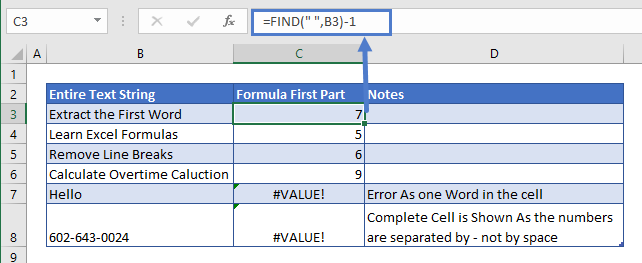### The LEFT Function

The LEFT Function will extract a certain number of characters from the string, starting from the left. Inputting the result from the FIND Function, gives us the first word:

``=LEFT(B3,C3)``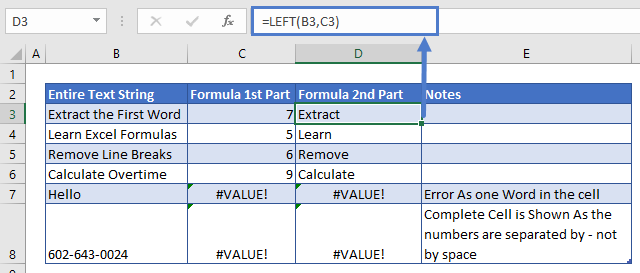## Error Handling

If the cell only has one word, the formula will return the #VALUE error.

So to prevent the formula from returning an error, we can use the following two options using:

### The IFERROR Function

Surround the formula with the IFERROR Function.

``=IFERROR(LEFT(B3,FIND("-",B3)-1),B3)``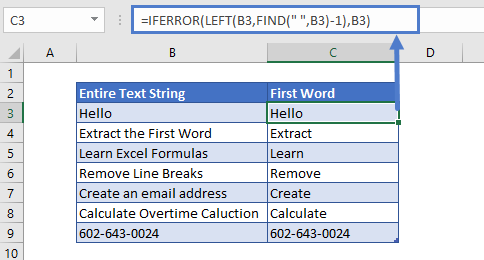The IFERROR function performs a calculation. If the calculation results in an error, it returns another value (or calculation). Above, the “iferror” value is the original text string.

### Simpler Way

Another way to prevent errors is by adding an additional space to the second argument of the FIND function, with ampersand operator, like this:

``=LEFT(B3,FIND(" ",B3&" ")-1)``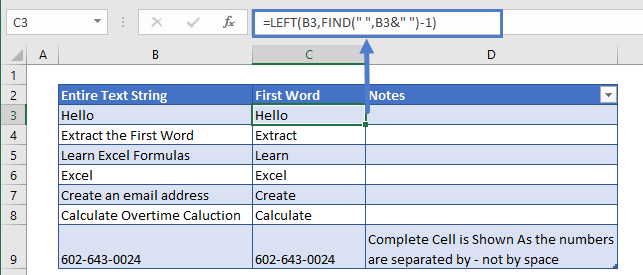Now the FIND function will always find a space because we’ve appended a space to the end of each string of text.

### Easy Excel Automation## Non-Space Separators

If the text string is not separated by space but from a different delimiting character, then by simply changing the value of the first argument in the FIND function with that delimiting character, we’ll get the first word from the text.

``=LEFT(B3,FIND("-",B3&" ")-1)``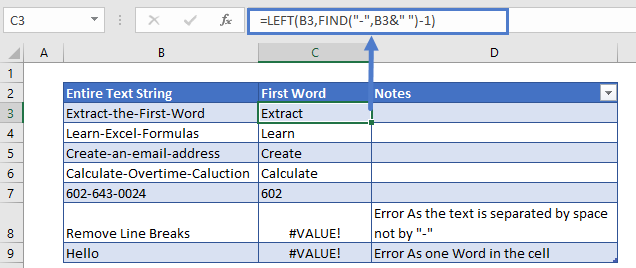## Get Last Word

Extracting the last word from a string of text is more complicated. One option is to use a formula like this:

``=TRIM(RIGHT(SUBSTITUTE(B3," ",REPT(" ",LEN(B3))),LEN(B3)))``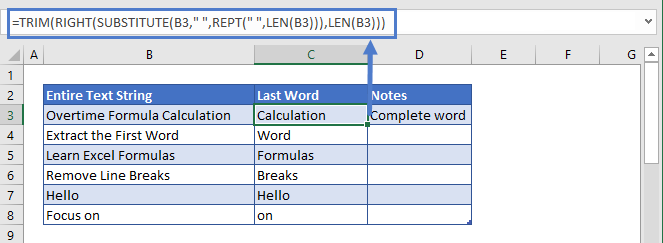## Extract First Word In Google Sheets

The formula to extract the first word from a text works exactly the same in Google Sheets as in Excel: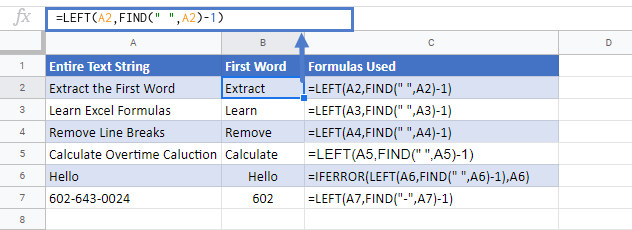### Excel Practice Worksheet

Practice Excel functions and formulas with our 100% free practice worksheets!